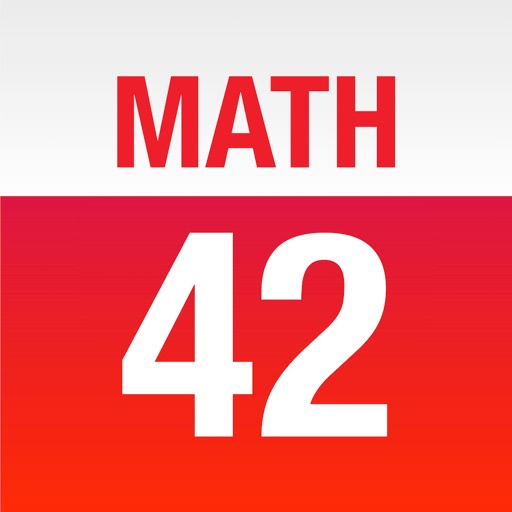## With over 3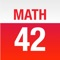# Math 42

by Chegg, Inc.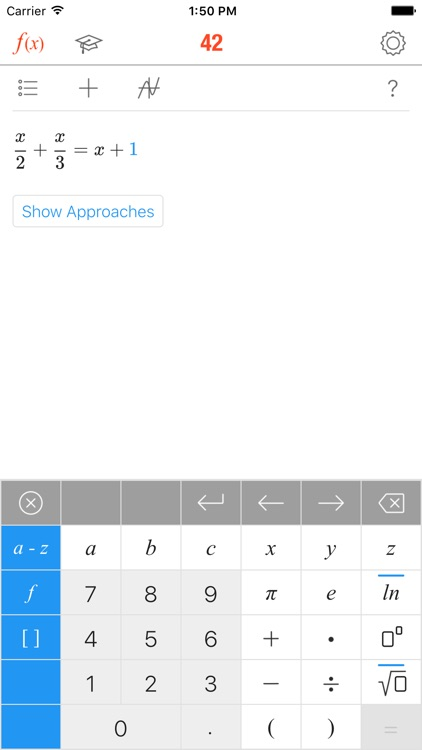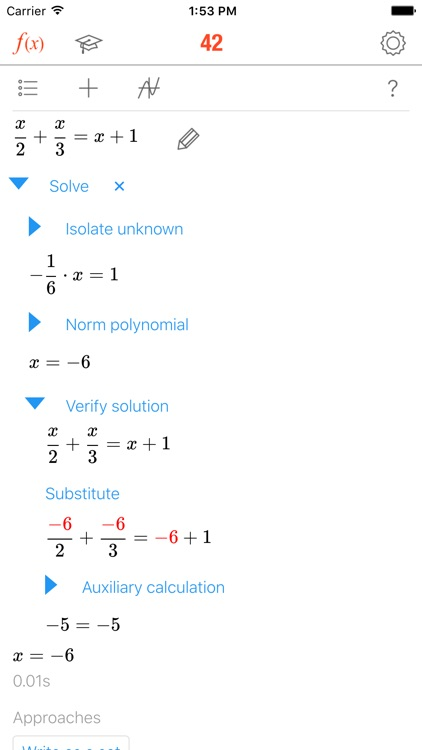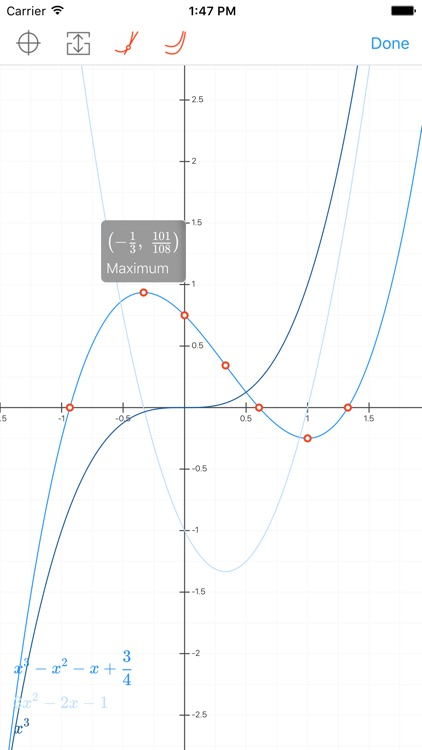FREE in the App Store

An all-in-one calculator that doubles as a learning tool.

With over 3.4 million downloads, MATH 42 is trusted worldwide by middle-school, high-school and college students to solve math problems, understand solutions, and get better grades. At a fraction of the cost of private tutors, MATH 42 delivers step-by-step solutions, intelligent approaches, and an Assessment Center for improving your skills.### App Details

Version
3.3.24
Rating
(303)
Size
73Mb
Genre
Education Productivity
Last updated
July 3, 2018
Release date
July 16, 2013

• \$2.99

• \$1.99

• \$11.99

• \$5.99

• \$7.99

### App Screenshots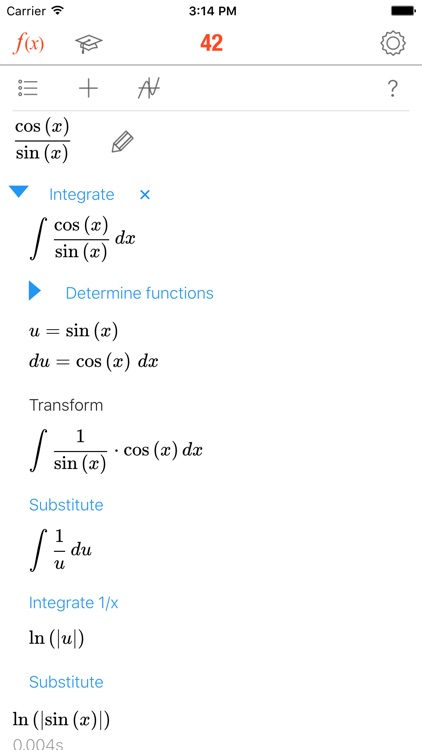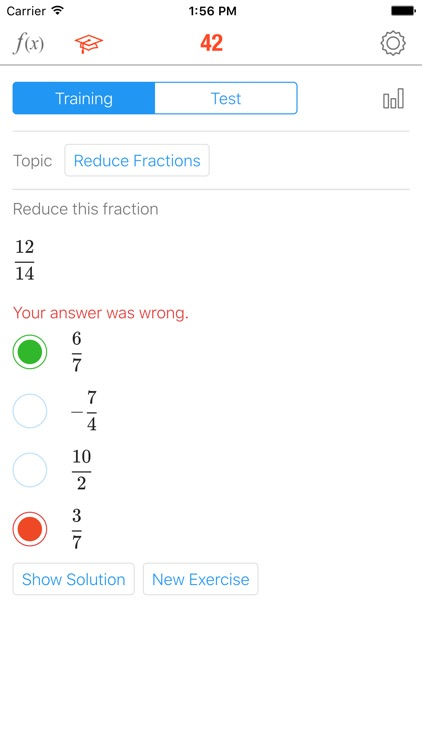### App Store Description

With over 3.4 million downloads, MATH 42 is trusted worldwide by middle-school, high-school and college students to solve math problems, understand solutions, and get better grades. At a fraction of the cost of private tutors, MATH 42 delivers step-by-step solutions, intelligent approaches, and an Assessment Center for improving your skills.

TECHNOLOGY
• MATH 42 works without templates or pre-calculated solutions
• MATH 42 solves all problems on the fly, like a teacher at the board
• MATH 42 is a real symbolic algebra system
• MATH 42 is based on artificial intelligence

FEATURES
• Intuitive formula entry
• Calculator that instantly computes during input
• Interactive step-by-step solutions
• Suggestions on how to approach a problem
• Explanations of relevant math concepts with examples
• Interactive graphs to help visualize problems
• Assessment Center that fuels progress with tests and exercises

ASSESSMENT CENTER
• Tests and exercises created with educators and private tutors
• Training mode in which each problem can be analyzed step-by-step
• Test mode to identify strengths and weaknesses
• Results and statistics of progress over time

CAPABILITIES
Term transformation
• Simplifying and expanding
• Factorization (factoring out, applying binomial formulas)
• Transforming powers and roots
• Adding, multiplying, reducing and expanding fractions
• Computing the gcd and the lcm
• Trigonometric transformations
• Logarithmic transformations

Functions
• Roots
• Y-axis intersection
• Domain of definition
• Extremes (maximums, minimums)
• Inflexion points
• Curve sketching
• Derivation
• Fundamental derivatives
• Factor rule
• Sum rule
• Product rule
• Quotient rule
• Chain rule
• Integration
• Fundamental integrals
• Integration by substitution
• Integration by parts
• Partial fraction decomposition

Equations
• Linear equations
• Special equations of higher degree
• Linear systems of equations
• Non-linear system of equations

Matrices
• Multiplying matrices
• Transposing matrices
• Inverting matrices
• Determinants
• Characteristic polynomials
• Eigenvalues
• Eigenspaces
• Kernels
• Ranks
• Cross-product
• Gaussian elimination

Disclaimer:
AppAdvice does not own this application and only provides images and links contained in the iTunes Search API, to help our users find the best apps to download. If you are the developer of this app and would like your information removed, please send a request to [email protected] and your information will be removed.